# Linear Functions and Slope Part A: Linear Relationships in Patterns (35 minutes)

## Session 5, Part A

### In This Part

• Finding the Pattern

### Finding the Pattern

A function expresses a relationship between variables. For example, consider the number of toothpicks needed to make a row of squares. The number of toothpicks needed depends on the number of squares we want to make. If we call the number of toothpicks T and the number of squares S, we could say that T is a function of S. S is called the independent variable in this case, and T the dependent variable — the value of T depends upon whatever we determine the value of S to be. Note 2

In this section, we’ll explore the dependence of one variable on another with the help of a spreadsheet program.

Make a row of squares using toothpicks. The squares are joined at the side.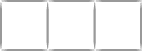### Problem A1

How many toothpicks are needed for one square? For two squares? For five squares? Make a table of these values.

 Squares Toothpicks 1 4 2 3 4 5

### Problem A2

Develop a formula describing the number of toothpicks as a function of the number of squares.

Tip: As in Session 2, try to develop the formula based on the context of the toothpick squares.

 Video Segment In this video segment, Gina explains her solution to Problem A2, including how she generated a rule for the number of toothpicks in each row of squares. Watch the segment after you have completed Problem A2. If you get stuck on the problem, the video segment may help you come up with a solution. Does Gina’s work involve a closed-form description or a recursive description for the number of toothpicks in each new row? Refer to Session 2, Part E for more information on these two kinds of descriptions. You can find this segment on the session video, approximately 3 minutes and 9 seconds after the Annenberg Media logo.

Problem A3

As you add each new square to the row, how many toothpicks are added? This gives you a recursive rule for the number of toothpicks.

Problems in Part A adapted from IMPACT Mathematics Course 1, developed by Education Development Center, Inc. (New York: Glencoe/McGraw-Hill, 2000), p.32.

Because of technology, spreadsheets have progressed from a handwritten accounting practice to a powerful tool in mathematics and modeling. A spreadsheet is a grid of cells, where each cell has a column label and a row label.

This section of Part A is designed for those who don’t have much, or any, experience using spreadsheets. If you are familiar with spreadsheets and spreadsheet software, please go on to the next section, “Using a Spreadsheet.”

• Cells
• Formulas
• Dynamic Change
• Filling Cells with Formulas
• Closed-Form and Recursive Rules
• Graphs

Cells

For the purposes of this tutorial, the column heading for a cell will be lettered, starting with A, and the row heading for a cell will be numbered, starting with 1. For example, the cell in the second column and third row would be cell B3. It is a common error to reverse these labels.

While a cell can only contain one piece of information, there are several types of information it can hold. To enter information into a cell, simply select that cell by clicking on it. A box will surround the selected cell at any time, and you can move to any surrounding cell by using the keyboard’s arrow keys. A cell can contain text, such as a word or a title, or numbers in many different formats (whole number, decimal, or dollars and cents, among others).

A cell can also contain a mathematical computation; this is typically done by placing an equal sign (“=”) in the cell followed by the computation, like this: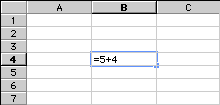When performing computations, the equal sign is very important; without it, a spreadsheet program may interpret the computation as text. Computers use certain characters for each operation, as follows:

Addition: use +. Typing “=6+7” in a cell will display the result, 13.
Subtraction: use -. Typing “=8-3” in a cell will display the result, 5.
Multiplication: use * (shift-8). Typing “=5*6” will display 30. Do not use the letter x for multiplication on a spreadsheet.
Division: use / (forward slash, to right of period). Typing “=12/3” will display 4.
Exponentiation: use ^ (shift-6). Typing “=4^3” will display 64.

Important: Do not type the quotation marks used in the examples! They are not part of any computation or formula. If you type quotation marks, the spreadsheet program will automatically interpret what you type as text, and will not perform any calculations.

Even though the result of the calculation is displayed, the spreadsheet remembers the details of the calculation. If you click on a cell, you can see the details of the calculation on screen.

Formulas

The power of a spreadsheet lies in its use of formulas. Each cell in a spreadsheet is able to contain its own formula. A formula in one cell can perform a calculation using values placed or calculated in other cells.

A demonstration here will be helpful in understanding this. Suppose you wanted to make a list of consecutive numbers. You could simply enter the value of each number in the cells, or you could create a formula to accomplish the same goal.

First, type the value 1 in cell A1. In cell A2, type “=A1+1” instead of typing the value. The use of A1 is a reference to cell A1, and the calculation will use whatever value was stored in cell A1. Since that value was 1, cell A2 will display “2”.

This can be continued in any other cell of the spreadsheet. Continue the list by entering into cell A3 “=A2+1”, and entering into cell A4 “=A3+1”. You should now see the numbers 1, 2, 3, and 4 listed in these cells.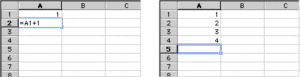Dynamic Change

Most spreadsheet programs include a feature that updates all values in the spreadsheet whenever one is changed. In the last example, cell A2 was defined to be 1 more than cell A1, cell A3 was defined as 1 more than cell A2, and cell A4 was defined as 1 more than cell A3.

Change the value in cell A1 to 23 and observe what happens.

Once a formula is entered, many values can be tested easily and quickly using this feature. Users can create a spreadsheet to model population growth, then instantly judge the long-term effects of a change. At home, this feature allows quick updating to a budget or a retirement plan. In many professions, the ability to use spreadsheets has become as important as the ability to use word processing software.

Filling Cells with Formulas

In the above example, you typed the same kind of formula several times.

Cell A2: “=A1+1”
Cell A3: “=A2+1”
Cell A4: “=A3+1”

All of these formulas can be interpreted as “Take the value in the cell above, and add 1.”

Nearly all spreadsheet programs allow the user to fill a formula over a group of cells, and the formula will adjust to the new location. To do this, the user must first highlight (select) a group of cells.

Click on cell A4 and hold down the mouse button. Now, drag the mouse down to cell A10. The cells from A5 to A10 should become darkened, while cell A4 remains selected.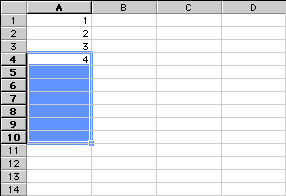Now, find the command “Fill Down” and select it. In a menu-driven spreadsheet, the Fill command is probably located in the Edit menu. The pattern you started in cells A1 through A4 should be continued through cell A10. More importantly, the formulas used in cells A2 through A4 should be continued, so that changing the value in cell A1 will change the entire spreadsheet. Change cell A1 back to the number 1 before proceeding.

Closed-Form and Recursive Rules

In Session 2, Part A we first encountered tables which could be described by several different rules.

A closed-form rule is a rule that describes how to take an input and directly determine an output. For example, the rule “take the input, multiply by 4, then add 2” is a closed-form rule. In a spreadsheet, a closed-form rule can be expressed in two columns: the left-hand column of inputs, and the right-hand column of outputs.

The rule just described could be expressed in a spreadsheet by entering the following formula into cell B1:

“=A1*4+2”

This formula takes the value in A1, multiplies it by 4, and then adds 2. To produce the information in other cells, fill down the formula from B1 to B10. In B2 you should have “=A2*4+2”, and so on.

A recursive rule is a rule that describes how to proceed from one input to the next. A recursive rule for the table of outputs we just created might be “The first output is 6; to get any other output, take the last output and add 4.” Think about how you could enter this rule into a spreadsheet in column C before revealing the solution tip below.

Tip: Enter the number 6 in cell C1, then enter “=C1+4” in cell C2. Finally, fill down the formula from cell C2 to C10. Important: Do not fill down from cell C1, since it does not contain a formula! If you filled down from cell C1, each entry in the column would be 6.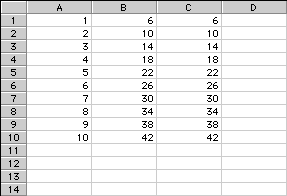Closed-form and recursive rules can work in tandem. There are many situations where one type of rule more easily describes a situation. We will be using both types of rules, so make sure you are comfortable using them both.

Graphs

While different spreadsheets create charts and graphs in their own way, most have an automatic graphing tool included. To create a graph, first highlight the cells you wish to use in the graph. On your spreadsheet, highlight cells A1 through B10 by clicking on A1, holding down the mouse button while you move the mouse, and releasing on cell B10.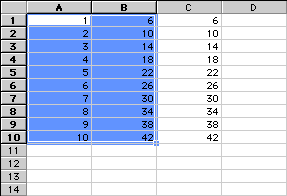Then find the graphing tool, which may be an icon at the top of the screen that looks like a chart. Selecting this will bring up a window asking what type of chart you would like to use; this choice will depend on the situation, but for this example, select a line graph. Be sure to select a graph that places the first column of information on the horizontal axis and the second column on the vertical axis. If you have done this correctly, the graph should be a straight line containing 10 points.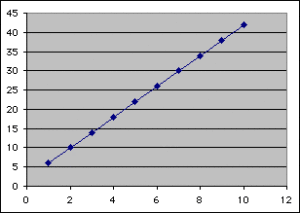Most graphing tools allow you to preview the graph before it is finalized, and you may need to try several options before the graph looks the way you want it to. If you have trouble with this feature, you should consult the help function or user manuals for your spreadsheet program.

Problem A4

Open a spreadsheet on your computer and enter your table from Problem A1 into the first two columns. Each cell in the first column will contain the number of squares in a row. The cell next to it in the second column will contain the number of toothpicks needed to build that number of squares. Note 3

Problem A5

Use your spreadsheet program to create a graph of the numbers in these two columns

 Video Segment In this video segment, Professor Cossey asks whether or not the points on Problem A5’s graph should be connected with a line. Watch the segment after you’ve completed Problem A5. In what situations should the points on a graph be connected? What does connecting two points on a line imply about the points in between? You can find this segment on the session video, approximately 7 minutes and 23 seconds after the Annenberg Media logo.

Different types of function rules can be entered into a spreadsheet. Note 4

Example: Closed-Form Rule
The formula y = 3x + 7 expresses the dependent variable y as a function of the independent variable x. Suppose that the values of x appear in the first column, beginning at cell A2, and the corresponding values of y are placed in the third column, beginning with C2. The formula would first be entered in C2 as “=3*A2+1,” and then the fill down command would be used to complete the remaining values of y.

Example: Recursive Rule
Suppose you have a recursive rule that says “The first output is 10; to get any other output, subtract 2 from the output before it.” You would enter the first output into cell C2. Then you could enter the formula “=C2-2” in C3, and use the fill down command for the remaining cells.

Problem A6

Enter your formula from Problem A2 into the third column of your spreadsheet. Compare the values computed by the spreadsheet program using your formula with those you entered by hand. Are they the same?

Problem A7

Enter your recursive rule from Problem A3 into the fourth column of your spreadsheet. Are the values computed by the spreadsheet program the same as those you entered by hand?

You can extend your formula to more values by filling down the output column. You will need to either enter the input numbers by hand or enter a formula that increases numbers in that column by one each time. See the spreadsheet tutorial for more information on how to do this.

Problem A8

Use the spreadsheet program to create a graph of the input/output columns for either of your rules.

### Notes

Note 2

This section begins with a variation of the toothpick problem from Session 2, allowing us to explore the concept of linearity in a familiar context.

Groups: It’s likely that people will have varying degrees of comfort and familiarity with spreadsheets, so working in groups may be helpful. Work on Problems A1-A3, completing the table and coming up with the rules. You may want to share rules before moving to the computers to work on the rest of Part A.

Note 3

The first use of the spreadsheet in Problem A4 requires only the entry of data. To draw the graph for Problem A5, select the data, and then select the graphing tool (this is called the Chart Wizard if you’re using Excel). The type of graph or chart for representing a functional relationship is an XY graph, or scatterplot. Some of the scatterplots will display only points, like this: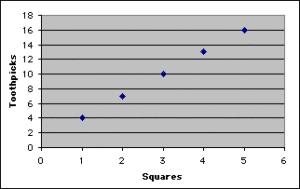Others will connect the points with a line: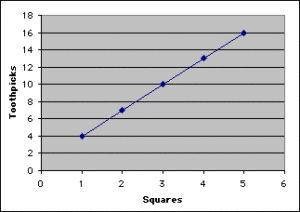Groups: After creating the graphs, pause to discuss which graph was chosen to represent the function. Some may say that the points should not be connected because, in this context, the function makes sense only for whole numbers. For example, it may not make sense to talk about how many squares 4.5 toothpicks can make. It is often useful, however, to connect the points on a graph like this so that we can see the shape of the function (in this case, a straight line) more clearly. This is often done by indicating the actual data points by large dots or diamonds and then connecting them with a narrower line.

Note 4

An important feature of spreadsheet programs is the ability to transfer formulas from one cell to another, updating cell references appropriately. This is called “filling,” and it is done most easily in Excel by selecting “Fill” and then “Down” from the Edit menu. Some may also know how to do this by dragging the formula from one cell to another. This method also works, but is hard to learn at first.

Once all four columns are filled, the spreadsheet should look something like this: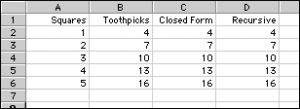It’s worth noting some pitfalls in working with spreadsheets:

• Forgetting the “=” at the beginning of a formula
• Beginning to type data before selecting a cell
• Expressing the cell coordinates in the wrong order — the letter for the column must precede the number for the row
• Forgetting to use the “*” for multiplication

End Part A by printing copies of the toothpick graphs or copying the graphs onto graph paper.

### Solutions

Problem A1

The first square requires 4 toothpicks. The second square requires 3 more, bringing the total to 7. Each additional square requires 3 more toothpicks. Here is the completed table:

 Squares Toothpicks 1 4 2 7 3 10 4 13 5 16

Problem A2

The formula is T = 3S + 1.

Problem A3

Three toothpicks are added each time, so a recursive description is that the nth square will require 3 more toothpicks than the one before it. A formula would be written Tn = Tn-1 + 3.

Problem A4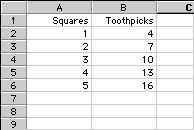Problem A5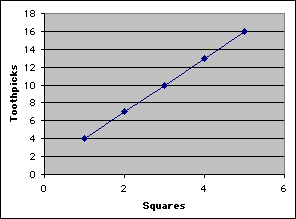Problem A6

If both rules describe the pattern correctly, the values computed by the spreadsheet should be the same as the values in the table. Note that the spreadsheet information in the columns is not the same; the third column contains formulas, and the screen shows the result of these formulas.

Problem A7

Again, the values should be the same, even though the formulas are not.

Problem A8

This should be the same graph as the one you found in Problem A5.